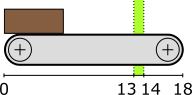## Algebraic variables

Consider a conveyor belt with a product on it:The product starts at the left side, at position `0`. There is a sensor that can detect the product between positions `13` and `14`. The product exits the conveyor at position `18`. The following CIF specification models the conveyor, product, and sensor:

``````automaton conveyor:
disc real position = 0.0;
event move;

const real width = 6;
alg bool sensor = position + width >= 13 and position <= 14;

location:
initial;
edge move when position < 18 do position := position + 0.1;
end``````

The `conveyor` automaton models the conveyor, with a product on it. The product is modeled by means of the `position` of the left side of the product, relative to the left side of the conveyor. As the conveyor starts to `move`, the product moves as well, and its position on the conveyor is updated. The product moves in steps of `0.1`.

The `width` of the product is `6`. The sensor is on whenever the product, which spans from `position` to `position + width`, is within the sensor range, which spans from `13` to `14`. An algebraic variable named `sensor` is used here, to represent the value of the sensor.

An algebraic variable is a variable whose value is determined by its definition. For the `sensor` variable, its value is determined from a calculation involving variable `position` and constant `width`. Unlike discrete variables, algebraic variables can not be assigned a new value. The value of algebraic variable `sensor` changes automatically as the value of discrete variable `position` changes. The value of algebraic variable `sensor` is `true` whenever the product is over the sensor, and it is `false` otherwise.

If we had modeled the value of the sensor as a discrete variable, we would have had to update the variable for every edge where the value of variable `position` is updated. In this example, that is only one edge. However, if the variable would have been updated on multiple edges, the sensor value would also have to be updated for all those edges. Furthermore, when adding another edge that updates the `position` variable, the edge needs to be adapted to also update the `sensor` discrete variable, which can easily be forgotten. Using an algebraic variable, the value computation needs to be specified only once, and no changes to its value are needed, as the value always remains consistent with its definition.

Algebraic variables can be used to give an expression (computations) a name, similar to how constants can be used to give fixed values a name. The benefits of using an algebraic variable are similar to the benefits of using constants. Both can be used to improve readability, and to make it easier to consistently change the model.

Algebraic variables can also be used as an abstraction. Consider the following extension of the specification:

``````automaton light:
event turn_on, turn_off;

location off:
initial;
edge turn_on  when     conveyor.sensor goto on;

location on:
edge turn_off when not conveyor.sensor goto off;
end``````

The idea is to have a light turn on when a product is detected by the sensor, and have it turn off when the sensor no longer detects the product. The algebraic variable `sensor` is used in the guard conditions of the `light` automaton, to determine when the light should be turned on or off.

In the example, the `light` automaton only uses the `sensor` variable from automaton `conveyor`. It does not matter how the value of that variable is defined. Currently, it is defined in terms of variable `position` and constant `width`. However, if the `conveyor` automaton were modeled differently, the expression that defines the value of the algebraic variable could be changed, without the need to change the use of the variable in automaton `light`.